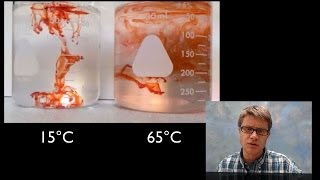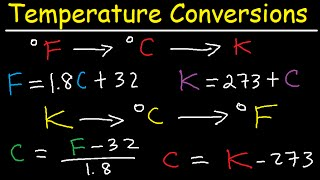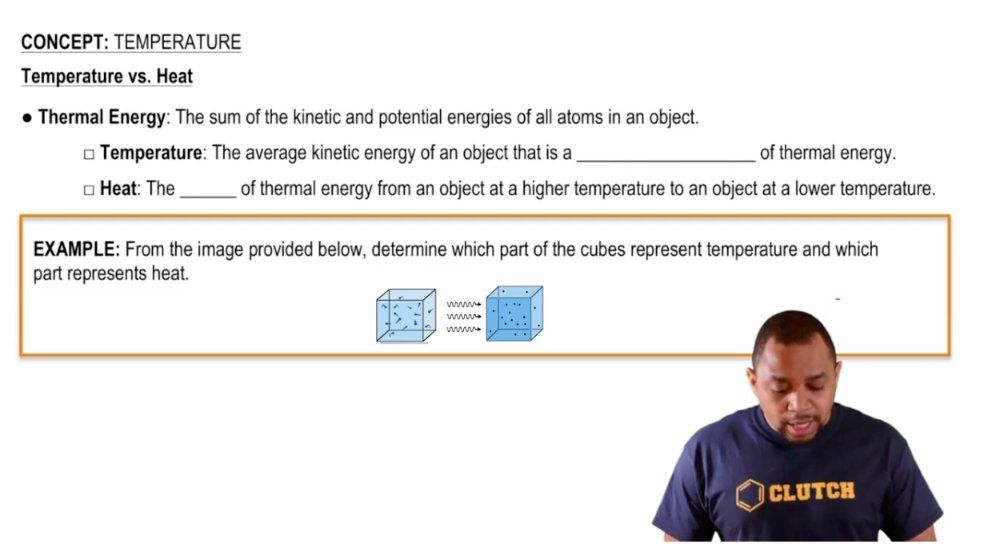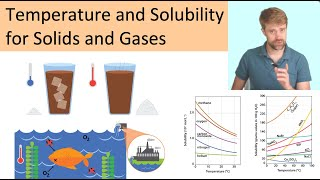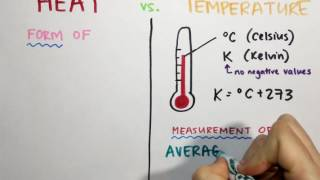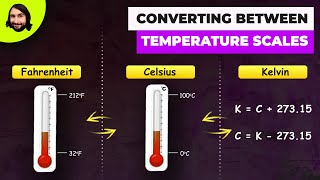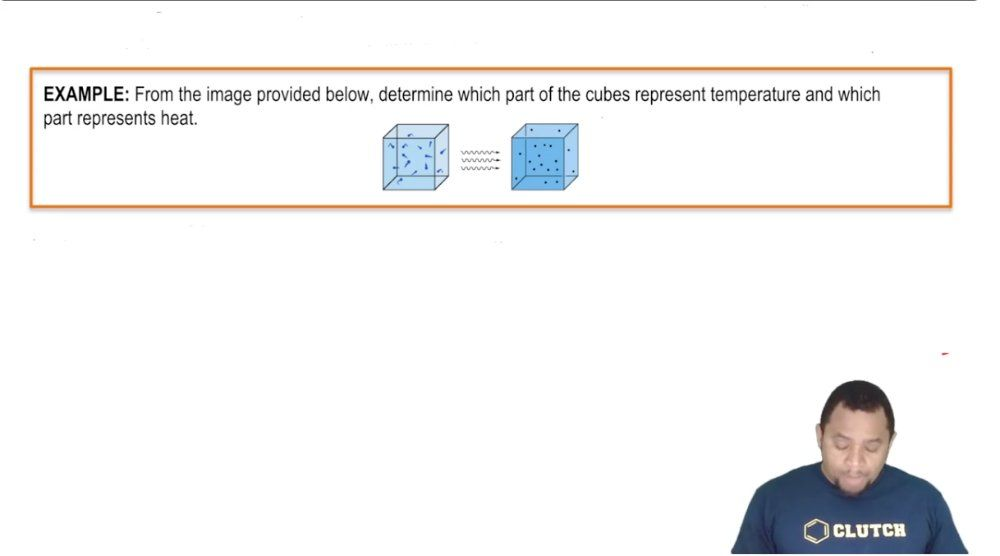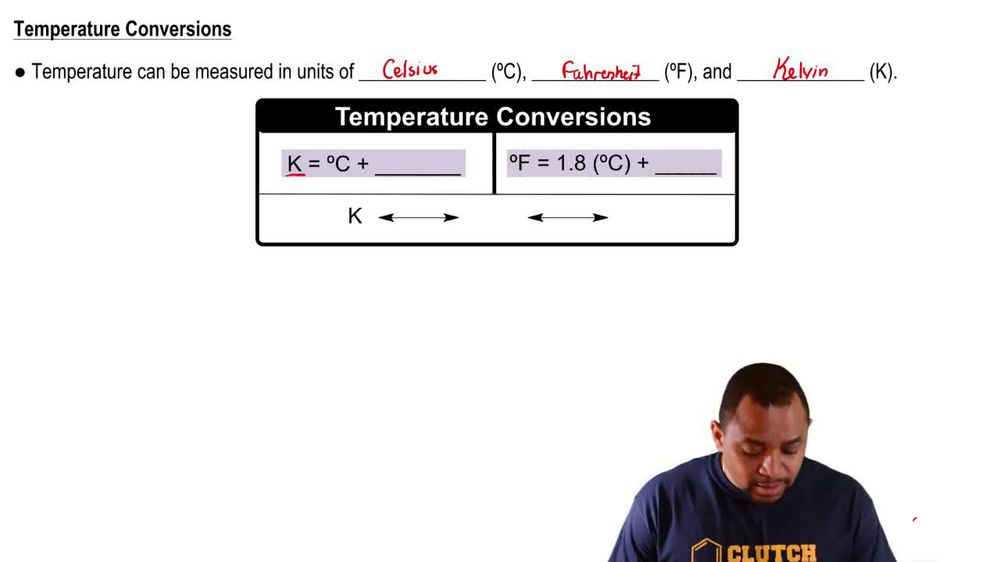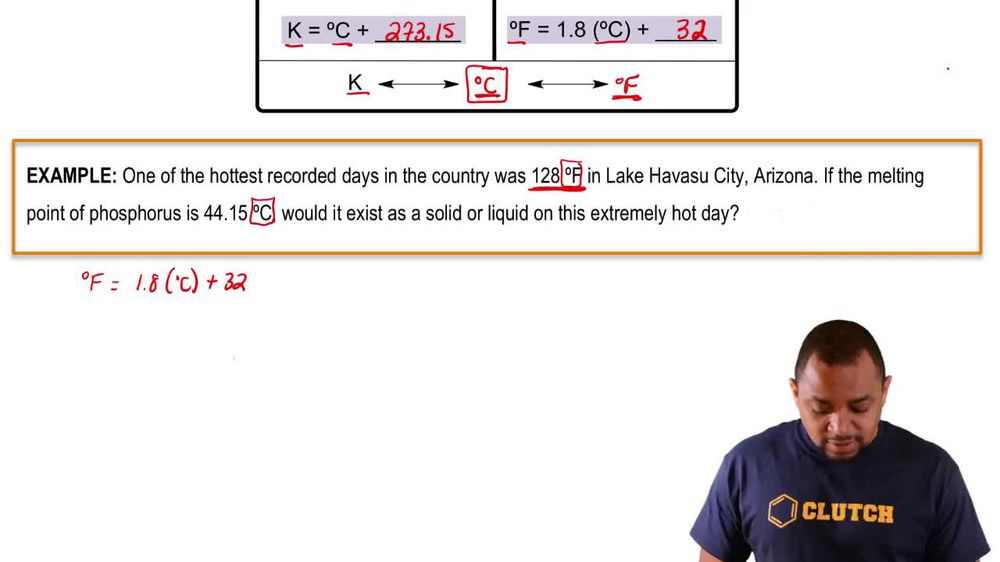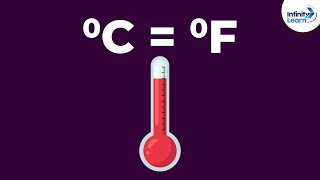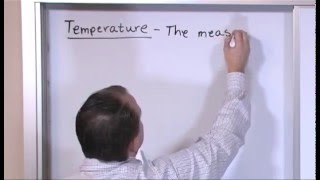Start typing, then use the up and down arrows to select an option from the list.
1. 1. Intro to General Chemistry2. Temperature# Temperature

by Jules Bruno
229 views
1
1
Now the words temperature and heat are often used interchangeably with one another, but they're not the same thing. They represent different aspects of thermal energy. Now, thermal energy itself is the sum of all kinetic and potential energies of all atoms in an object. Now, when we say kinetic energy, we know that kinetic energy is the energy of motion. Okay, so the movement of molecules, the movement of atoms, potential energy is just the energy of position. Where those molecules are related. Are they 100 ft off the ground? Are they 10 ft off the ground? Where exactly are they located? Now? Temperature, heat again are used interchangeably with one another, but they're not the same thing. Temperature itself is the average kinetic energy of an object. That is a measurement of thermal energy. Remember that temperature itself is an intensive property. It is the inside or innate properties of a substance that is independent on the amount of that substance. For example, if we have a cup of water that's 100 degrees Celsius versus a gallon of water, that's 100 degrees Celsius. The amount of water is different, but the temperature is the same because the temperatures the waters are are at at the moment, doesn't matter how much of the water we have. Okay, so temperature is an intensive property. Heat on the other hand, is not heat is the flow Of thermal energy from the object at a higher temperature to an object at a lower temperature. Okay, so it's normal for heat to move from a hotter object to a colder object. If you have a hotter object next to a colder object, eventually all the heat from the hotter object moves towards the colder one. So to heat it up, heat itself is based on the amount of the substance present. So it would represent an extensive property. Now, knowing the difference between temperature and heat is critical for the example, that's left right below it. If we take a look at this example, question it says from the image provided below, determine which part of the cubes represent temperature and which part represents heat. Alright, so we have two cubes here and we can see that the cube on the left has those little red balls that seem to be bouncing everywhere. They're highly energetic. That happens because the overall temperature in this cube is higher. So we say that this cube has a higher temperature. Then if we look on the other side we see that they are kind of like stuck in place not moving at the same speed as before. That's because there are a lower temperature. And remember we just said that heat goes from a higher temperature to a lower temperature object. That is explained by these wavy lines. So here we see that heat is leaving the left Cuban going to the right cube. So these wavy lines represent heat. So that's how you need to understand temperature is an actual measurement, 100 20 98 F. Uh These are actual numbers. Heat is just a flow of of of thermal energy from a hotter object to a colder object, although they're used interchangeably. Sometimes they are not the same thing now that we've talked about the difference between intensive when it comes to temperature and extensive when it comes to heat. Let's move on to our next practice question.